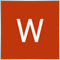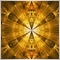# Trying to calculate total profit for trades - page 29153

okay you want to see all buy/sell profit of open orders?

set terminal to 'All History'

call function

`OrdersTotalInfo()`

reference global variables

`SellsOpenProfit, BuysOpenProfit`9153

the main reason i say it should help with profit & loss would because you can check each order open and close price, lot value as well as commission and swap and add up the totals for all buys and sells9153

jtubbs13791:
Thanks for the the help. it works, but it only pulls the info from the last open trade. Is there away to get all the open trades? Plus I am confused on how to get the closed profit amount. I know you change MODE_TRADES to MODE_HISTORY, but I am not getting any info. As you can tell I am fairly new to the coding scene.

heres what i do to find my pips for the day

```string _symbol=Symbol();
int newMagic=1234;
for(int GFTDPips=OrdersTotal()-1; GFTDPips>=0; GFTDPips--)
{
{
if(TimeDayOfYear(OrderOpenTime())==TimeDayOfYear(TimeCurrent()) &&
OrderSymbol()==_symbol && OrderMagicNumber()==newMagic && OrderComment()==_comment)
{
if(OrderType()==OP_SELL){_sellspips+=OrderOpenPrice()-OrderClosePrice();}
}
}
}
for(GFTDPips=OrdersHistoryTotal()-1; GFTDPips>=0; GFTDPips--)
{
OrderSelect(GFTDPips,SELECT_BY_POS,MODE_HISTORY);
{
if(TimeDayOfYear(OrderOpenTime())==TimeDayOfYear(TimeCurrent()) &&
OrderSymbol()==_symbol && OrderMagicNumber()==newMagic && OrderComment()==_comment)
{
if(OrderType()==OP_SELL){_sellspips+=OrderOpenPrice()-OrderClosePrice();}
}
}
}```22458

GumRai: Use iPos--
1. For POD types, ++x and x++ are equivalent.
2. For classes ++x is operator++(){ whatEver; return GetPointer(this) ; }
3. For classes x++ is operator++(int){ CLASS tmp(this); ++this ; return tmp;} which is more than twice as expensive.
4. Prefer ++x over x++80

```//Yesterday Profit
double YesterdayProfit(){
datetime yesterday = TimeCurrent() - 60 * 60 * 24;
string time_yyyy_MM_dd = TimeToString(yesterday, TIME_DATE);
double profit = 0;
for(int i = 0; i < OrdersHistoryTotal(); i++){
if(OrderSelect(i, SELECT_BY_POS, MODE_HISTORY)){
if(time_yyyy_MM_dd == TimeToStr(OrderCloseTime(), TIME_DATE)){
profit += OrderProfit() + OrderSwap() + OrderCommission();
}
}
}
return(profit);
}

//TodayProfit
double TodayProfit(){
double profit = 0;
for(int j = 0; j<OrdersHistoryTotal(); j++){
if(OrderSelect(j, SELECT_BY_POS, MODE_HISTORY) == TRUE){
if(OrderType() > 1){
continue;
}
if(TimeToStr(TimeCurrent(), TIME_DATE) == TimeToStr(OrderCloseTime(), TIME_DATE)){
profit += OrderProfit() + OrderSwap() + OrderCommission();
}
}
}
return(profit);
}```12

i m finding problem in closing a trade with profit...can someone plz throw a code on it